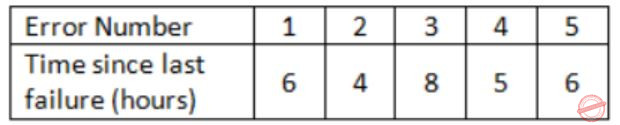October 5, 2023
October 5, 2023
October 5, 2023
###### Software-Engineering
October 5, 2023
 Question 15

The following table shoes the time between failures for a software systemThe reliability of the system for one hour of operation assuming an exponential model is

 A 0.45 B 0.63 C 0.84 D 0.95
Question 15 Explanation:
MIBF = ∑(Start of downtime – Start of uptime)/No. of failures
MIBF = (6+4+8+5+6)/5 = 29/5
The probability or reliability that the product will work for a defined period of time without failure is given by
R(T) = exp(-T/MTBF); T = 1 hour
R(1) = e(-1/(29/5)) = e(-5/29) = 0.84
Question 15 Explanation:
MIBF = ∑(Start of downtime – Start of uptime)/No. of failures
MIBF = (6+4+8+5+6)/5 = 29/5
The probability or reliability that the product will work for a defined period of time without failure is given by
R(T) = exp(-T/MTBF); T = 1 hour
R(1) = e(-1/(29/5)) = e(-5/29) = 0.84